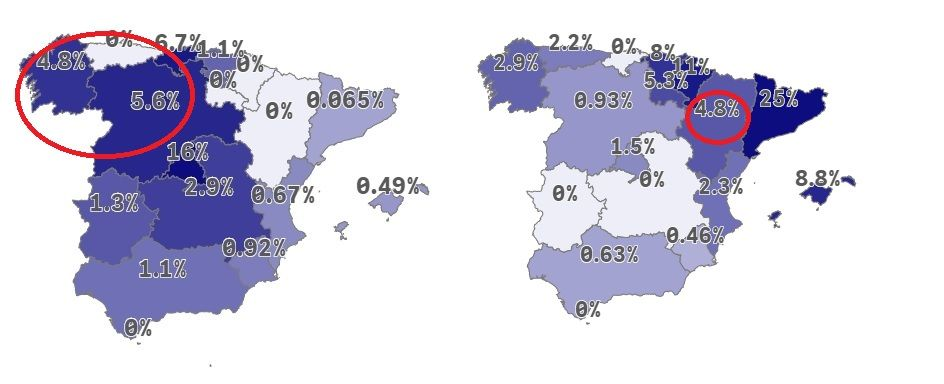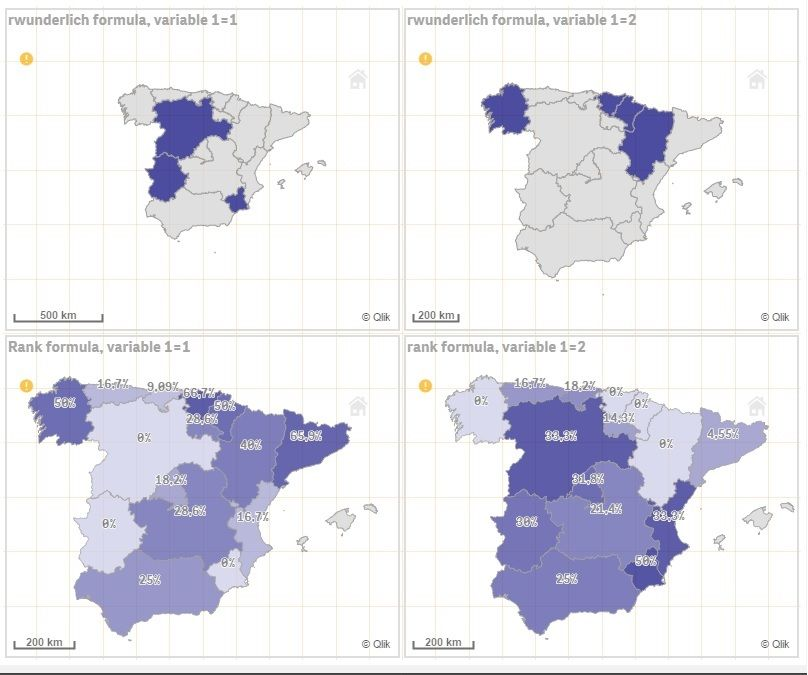# New to Qlik Sense

Discussion board where members can get started with Qlik Sense.

Announcements
Talk to Experts, a LIVE Q&A Webinar. Bring your Qlik Sense Business questions on Aug. 4th. Register
cancel
Showing results for
Did you mean:
HighlightedCreator

## Colormix function Min and Max Value setting

Hi,

I have problem when using the ColorMix function, the applications I have seen in the forum seems working good when one is fine with the Min and Max value that results from the variable applied.

The problem comes when I  apply this method to more than one map, it works as expected on each one  but I need that color scale is stable acrross all maps.

I see that the problem comes from the Min and the Max value on the ColorMix is adapted to the data set and variable on each map independently.

On the maps below, the darkest color on the left does not match the darkest on the right, and need them to have the same patterns in terms of color.Can I for example stablish a max value and min value by default acrross different maps and then so the colors are more or les stable?

This is the formula applied on the Left map, and it is quite similar to the one on the right (just setting [Variable_1] to {"2"})

ColorMix1(
rank(TOTAL

num(

count({<[Variable_1]={"1"}}>}distinct ID_PERSON)
/
count(distinct ID_PERSON)

,
'#,#%'
)

)/NoOfRows(TOTAL) ,
rgb(0,0,120)
,
rgb(250,250,255)

)

Labels (2)

• ### colormix2

7 Replies
HighlightedMVP & Luminary

## Re: Colormix function Min and Max Value setting

I think you could synchronize the two maps with something like this:

colormix((exp1 + exp2) / 2, color1, color2)

- Marcus

HighlightedCreator

## Re: Colormix function Min and Max Value setting

Thanks Marcus,

But that did not produce the expected result, in that way both maps have the same color on each province. When indeed the label to display is actually different in each map.

This solution provided produce a blend from both Expr1 and  Expre2. But I just need that if 5% is dark blue with an specific RGB value or whatever this logic is the same both maps, so one see the color from both maps simultanously the meaning of the color is the same in both.

HighlightedMVP & Luminary

## Re: Colormix function Min and Max Value setting

Your problem comes about when you convert that base value to a relative ranking, as a way to force the use of the entire color range.  But 5% (.05) would not have the same ranking across the two charts, hence the different colors.

How about computing the ratio of the max value to 100 and using that as a multiplier?

=100  /
RangeMax(
Aggr(count({<[Variable_1]={"1"}}>}distinct ID_PERSON) /count(distinct ID_PERSON), Country)
Aggr(count({<[Variable_1]={"2"}}>}distinct ID_PERSON) /count(distinct ID_PERSON), Country)
)

You could put that in a variable "vRatio" and then multiply by your base calculation.

ColorMix1(Column(n) * vRatio, rgb(0,0,120), rgb(250,250,255))

HighlightedCreator

## Re: Colormix function Min and Max Value setting

Hi,

Thanks for your answer. There might be something missing on the V_RATIO variable, maybe a "colon" , after "...country)"?

=100  /
RangeMax(
Aggr(count({<[Variable_1]={"1"}}>}distinct ID_PERSON) /count(distinct ID_PERSON), Country)
Aggr(count({<[Variable_1]={"2"}}>}distinct ID_PERSON) /count(distinct ID_PERSON), Country)
)

On the other hand, what means here Column(n) in the colormix function. The formula is supossed to be applied in the map layer color setting  of a qliksense map, or there is something I am not getting?

Luis

HighlightedMVP & Luminary

## Re: Colormix function Min and Max Value setting

I didn't test the expression. Looks like I missed "," (comma) after the first Country.

Column(n) is a shorthand way of referring to the value at measure number "n".  It's probably column(1) in a map.  The idea is to avoid repeating a expression you've already calculated. It's a little less clear in a map, you can use your original expression as well.

-Rob

HighlightedCreator

## Re: Colormix function Min and Max Value setting

Hi @rwunderlich  I tried your formula, but it didn´t produce the expected result.

ColorMix1(

(count({<[Variable_1]={"1"}}>}distinct ID_PERSON)
/
count(distinct ID_PERSON))

*

(100  /
RangeMax(
Aggr(count({<[Variable_1]={"1"}>}distinct ID_PERSON) /count(distinct ID_PERSON), Country),
Aggr(count({<[Variable_1]={"2"}>}distinct ID_PERSON) /count(distinct ID_PERSON), Country)
))

, rgb(0,0,120), rgb(250,250,255))

HighlightedCreator

## Re: Colormix function Min and Max Value setting

I have created an APP with the example of your formula, and also the formula that uses Rank (TOTAL expresion1) )/NoOfRows(TOTAL)

https://we.tl/t-PAz3hwXwJq